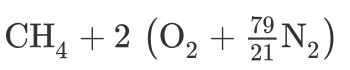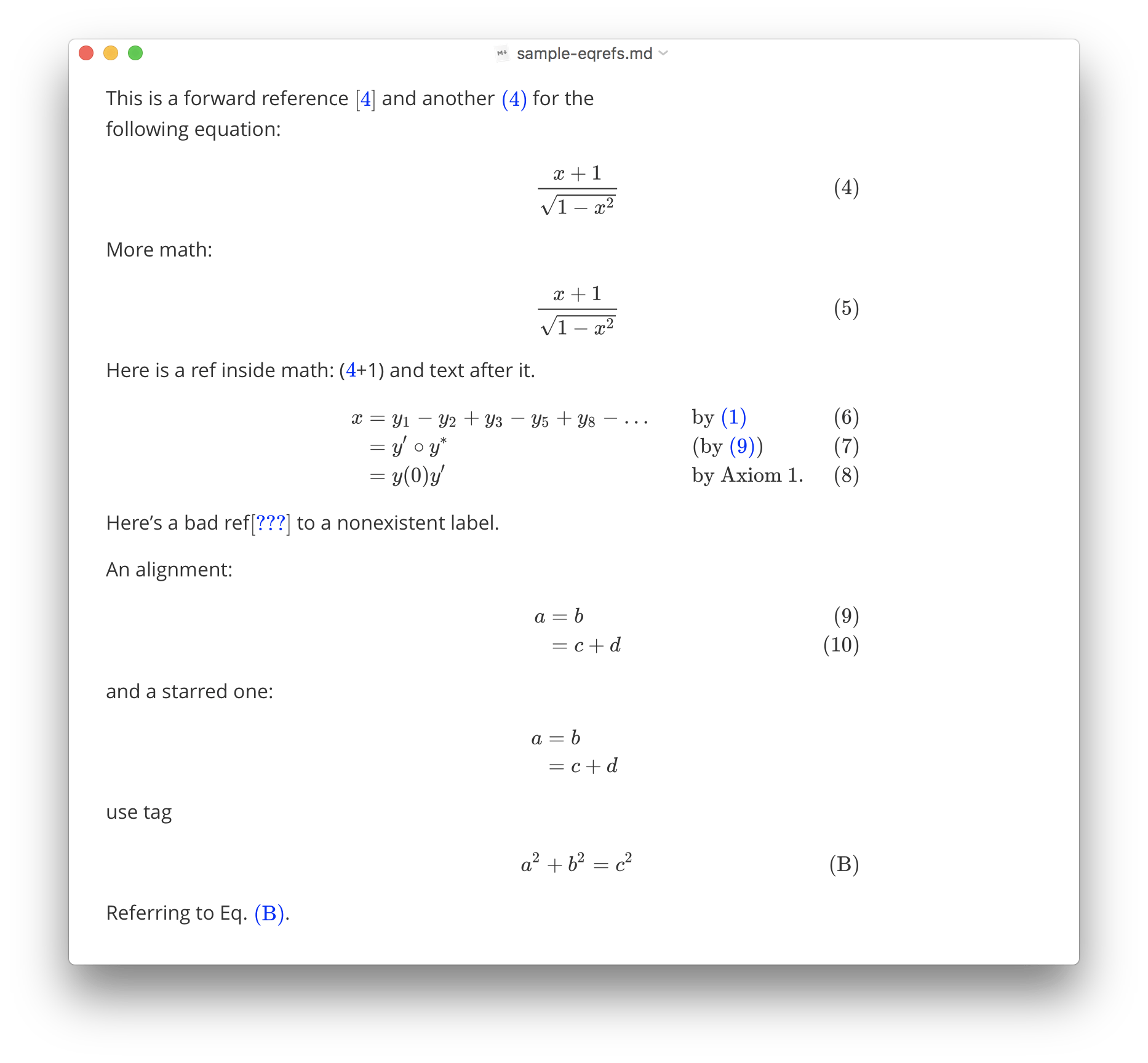Typora supports rendering normal mathematics using Tex/LaTeX syntax. The rendering process is processed by MathJax.

## Math Block (Display Math)

Math blocks are LaTeX expressions wrapped by $$ mark and line break, for example: $$
\begin{align*}
y = y(x,t) &= A e^{i\theta} \\
&= A (\cos \theta + i \sin \theta) \\
&= A (\cos(kx - \omega t) + i \sin(kx - \omega t)) \\
&= A\cos(kx - \omega t) + i A\sin(kx - \omega t)  \\
&= A\cos \Big(\frac{2\pi}{\lambda}x - \frac{2\pi v}{\lambda} t \Big) + i A\sin \Big(\frac{2\pi}{\lambda}x - \frac{2\pi v}{\lambda} t \Big)  \\
&= A\cos \frac{2\pi}{\lambda} (x - v t) + i A\sin \frac{2\pi}{\lambda} (x - v t)
\end{align*}
$$ will be rendered as In typora, you could just press $$ and Return key to input a math block, in input mode, use Up/Down arrow key or Command/Ctrl + Return key to finish editing, or just click the ✓ button, or somewhere else.

## Inline Math

First of all, please enable inline math feature from preferences panels -> Markdown section. Preferences panel can be opened From menu bar: File -> Preferences..., or use shortcut key — command/ctrl + ,. This setting will be applied after Typora restarts.Inline math use syntax like this $<Math Expressions>$, e.g: f = \frac{2 \pi}{T}$. ## TeX Commands available in Typora You could find the all supported TeX commands in http://docs.mathjax.org/en/latest/tex.html#supported-latex-commands. You could add new commands using \def or\newcommand. For example: $$\def\bold#1 \bold{this\ is\ now\ bold}$$  ## Chemistry Expressions Typora has the built-in mhchem extension, which can be used to render Chemistry Expressions, you could use like this: $\ce{CH4 + 2 $\left( \ce{O2 + 79/21 N2} \right)$}$ Which will be rendered asFor more details, please refer https://mhchem.github.io/MathJax-mhchem/. ## Cross Reference Typora supports TeX-style reference syntax, for example: Here is a labeled equation: $$x+1\over\sqrt{1-x^2}\label{ref1}$$ This is a referece :$\ref{ref1}\$


## Auto Numbering

Typora supports auto-numbering math blocks. To turn on this feature, please open preferences panel, and enable “Auto Numbering Math Equations” under the “Markdown” section.## Troubleshooting

### Force Refresh

When Math rendering goes wrong, like output math too wild/narrow, or equation numbering becomes incorrect, you can trigger force refresh for all math from the EditMath Tools menu.